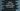# Python program to count total repeating digits in a number## Python program to count total repeating digits in a number:

In this post, we will learn how to count the total number of repeating digits in a given number. For example, for the number 87867, 7 and 8 are repeating. So, the answer is 2.

We can solve this problem in different ways. We can either use two for loops and find the value by iterating through the digits of the number or we can use a hashmap.

In this post, I will show you both of these ways.

### Method 1: By using two loops:

In this approach, we will use two for loops. Both loops will traverse the digits of the numbers from left to right. Then, for each digit, we will compare it with other digits in the number.

If any other digit equal to the current digit is found, i.e. the current digit is a duplicate digit. We can use the same approach for all other digits to find the total count of repeating digits in that number.

### Method 2: By using hashing:

Another, and an efficient way is to use a dictionary. We can follow the below steps to get the count:

• Initialize one empty dictionary.
• Iterate through the digits of the number one by one.
• For each digit, check if any key equal to it exists in the dictionary or not. If it doesn’t exists, add it with a value of 1. If it exists, increment its value by 1.
• The dictionary will hold the count of each digit of the number once each digits are checked.
• Once the iteration is completed, check the value of each key in the dictionary. If any value is more than 1, that is a repeated value. Calculate the total repeated values and print the result.

Below is the complete program:

``````def find_repeated_count(given_no):
counter_dict = {}

while given_no > 0:
right_digit = given_no % 10

if right_digit in counter_dict:
counter_dict[right_digit] = counter_dict[right_digit] + 1
else:
counter_dict[right_digit] = 1

given_no = int(given_no/10)

total = 0

for key in counter_dict:
if counter_dict[key] > 1:
total += 1

no = int(input('Enter a number :'))

print('Total repeated numbers: {}'.format(find_repeated_count(no)))``````

Here,

• find_repeated_count method is used to find the count of all repeated digits in a number.
• counter_dict is an empty dictionary.
• The while loop is used to iterate through the digits of the number one by one. It picks the rightmost digit of the number and then removes that digit from the number.
• The if-else block checks if any key equal to the current iterating digit is in the dictionary or not. If it is not, it adds that key with a value 1. Else, it increments the current value for that key by 1.
• The for in loop iterates through the keys of the dictionary and finds the total number of keys with value more than 1.
• It returns the total count it finds in the for-in loop.